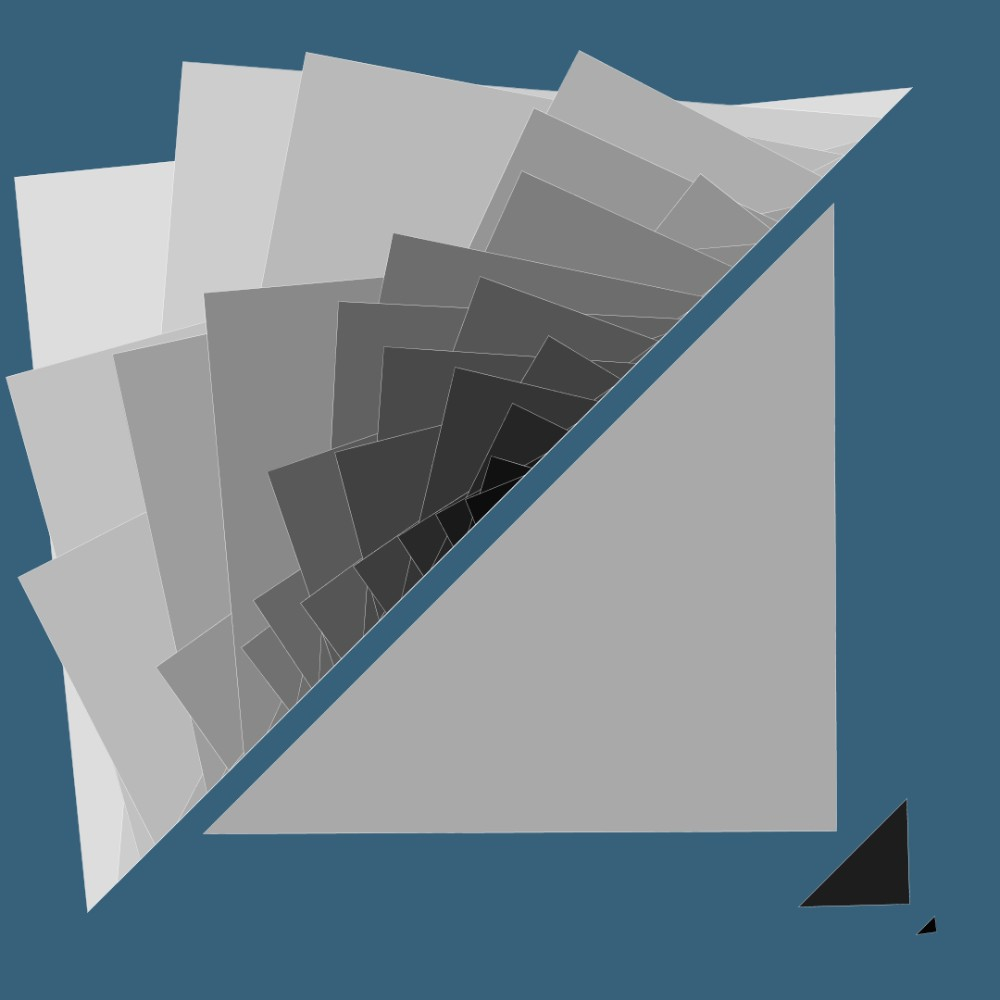Triples Digital Print 2016

Illustrated are primitive Pythagorean triangles with side leg length less than the arbitrary constant 180 and drawn with the hypotenuses of all triangles parallel to the main diagonal. The three triangles, (3, 4, 5), (20, 21, 29), and (119, 120, 169), shown below the main diagonal are almost-isosceles (their leg lengths differ by 1); the remaining triangles are shown above the main diagonal.

### Background and Inspiration

This work was originally done for use as cover art for the April 2016 issue of Mathematics Magazine. This is the seventh of 25 original artworks I created for the journal Mathematics Magazine during 2015–2019.

A Pythagorean triple $$(a, b, c)$$ of positive integers such that $$a^2 + b^2 = c^2$$. There are an infinite number of solutions, including $$(3, 4, 5)$$ and $$(5, 12, 13)$$. A right triangle can be constructed with legs of lengths $$a$$ and $$b$$ with a hypotenuse of length $$c$$. A Pythagorean triples is said to be primitive if $$a$$, $$b$$, and $$c$$ share no common factor greater than 1. There are no isosceles triangles with a corresponding Pythagorean triple ($$a$$ is never equal to $$b$$). However, Nelson (2016) showed there are infinitely many almost-isosceles Pythagorean triples, where $$|a - b| = 1$$. In general, polynomial equations where only integer solutions are sought are called Diophntine equations, named in honor of Diophantus of Alexandria.

### Publication History

• Mathematics Magazine, Cover Art, Vol. 89, No. 2, April 2016.

### References

• The Proof Without Words Infinitely Many Almost-Isosceles Pythagorean Triples Exist by Roger Nelsen was the inspiration for this piece.